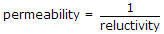# Electronics and Communication Engineering - Electromagnetic Field Theory

21.
Which one is not correct for folded dipole antenna?
High I/P impedance
Low I/P impedance
High bandwidth
Used as reactance compensation network
Explanation:
No answer description is available. Let's discuss.

22.
For VLF antennas radiation resistance is usually of the order of
less than one ohm
13 to 20 ohms
around 100 ohms
more than 200 ohms
Explanation:
No answer description is available. Let's discuss.

23.
The attenuation factor in parallel plane guides is given by
a = power lost/power transmitted
a = 2 x power lost/power transmitted
a = power lost per unit length/2 x power transmitted
a is always constant
Explanation:
No answer description is available. Let's discuss.

24.
Which of the following relations is incorrect?
mmf x reluctance = 1
conductance x resistance = 1none of the above